# Quiz 11: Pure Competition in Therun

We are given a payoff matrix of firm profits, depending on whether the firm is setting a high or low price: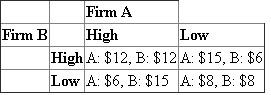The each cell in the above table denotes the payoff for the firm for the corresponding actions taken by both the firms. For example, if both Firm A and Firm B charges a high price, then both will get \$12 of profit. Whereas if Firm A charges a low price and Firm B charges a high price, then Firm A will get \$15 of profit and Firm B will get \$6. Suppose now a firm setting a low price will be fined by a firm charging a high price. Let's denote that fine by the variable "X". Then, we will have the following payoff table: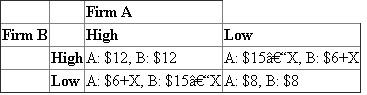Note that if both firms are charging high or both firms are charging low, it will not change anything. However, if Firm A charges a low price but Firm B charges a high price, Firm A will get fined \$X, which means their profit is now \$15-X, and Firm B will get the \$X fine as profit, and their profit is now \$6+X. We want to find the smallest value of X such that both firms will always set the high price. We see that currently, both firms will want to charge a low price. Let's look first at Firm B. Let's assume that Firm B thinks Firm A will choose a high price. Then, without the punishment, we see that Firm B will want to choose the low price, because it gives them a higher profit (\$15 compared to \$12 if they chose high, assuming Firm A chooses high). Suppose Firm B now thinks Firm A is going to choose a low price, in this case, their best response would also be to price low, since they will get \$8 of profit instead of \$6 of profit (if they choose to price high when the other firm chooses to price low). This is the same for Firm A as well, that they will choose to price low regardless of what Firm B does. Therefore, to change this, we must change the payoff such that choose to price high will generate a higher profit. With the added punishment element, we see that if X=\$3 (the fine is \$3), then this means that if Firm B thinks that Firm A will choose to price high, they are now choosing between \$12 of profit in pricing high or \$15-\$3=\$12 of profit if they choose to price low. Here, they are indifferent between choosing high or low. However, if they assume that Firm A will choose to price low, then they are now choosing between \$6+\$3=\$9 of profit in choosing to price high or \$8 of profit in pricing low. Here, they will want to price high instead. As they are indifferent between pricing high or low when Firm A prices high but would want to price high if Firm B prices low, they will choose to price high. Since Firm A's problem is symmetric, they will also want to price high. Therefore, the smallest value such that both firms will want to price high is X=\$3.

The following payoff matrix represents one combination of a RareAir strategy and an Uptown Strategy and shows the profit that combination would earn for each.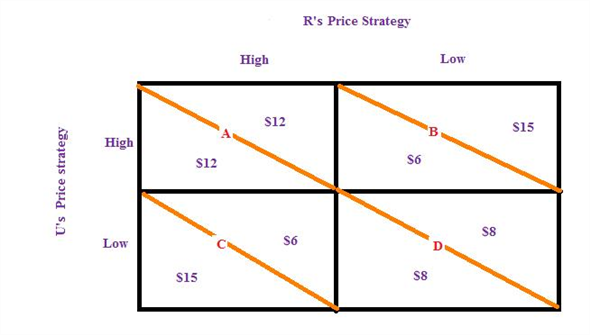The above game is a positive-sum game because the sum of the outcomes (i.e. profits) of the two firms' is positive; here both firms have positive outcomes (i.e. profits). In cell A the sum of the profits of the two firms are \$(12+12) = \$24 Yes, there are dominant strategies for the both the firms. For Uptown firm the dominant strategy is low price strategy because the firm will adopt this strategy irrespective of what RareAir does. It is due to the fact that the profit of Uptown will be high with low price strategy if RareAir chooses either a high price strategy (\$15 in place of \$12 profit for Uptown) or RareAir chooses either a low price strategy (\$8 in place of \$6 profit for Uptown). Similarly, low price is a dominant strategy for RareAir. Cell D (i.e. \$8 profit to each firm) represents a Nash equilibrium because in this case both firms adopt their dominant strategies simultaneously, hence it is an outcome from which neither rival wants to deviate. It is difficult to achieve and maintain a more favorable cell than the Nash equilibrium in this single-period pricing game because if either of the two firms tries to deviate from their dominant strategy then the game would temporarily move to either cell B or cell C. But once discovered, the game would return to the Nash equilibrium. Hence, neither rival would deviate from the Nash equilibrium.

Recall that the four-firm concentration ratio is defined as: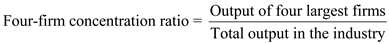Since we are given already the shares of the local hamburger market, so, we do not need to find the actual output of each firm and the total output of the industry, but we only need to use the percentages that are given. The largest four firms have market shares of 23%, 22%, 18%, anD12%. Therefore, the four largest firms together take up 75% of the local burger market. Since the total output in the industry iS100%, dividing 75% by 100% gives us.75, which is the four-firm concentration ratio of the burger industry. We know that the Herfindahl index is defined as: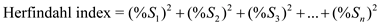, with S1 , S2 , …, S n denoting the percentage market share of firmS1, 2, …, n, respectively. Then, substituting in the market shares of each of the seven burger shops, we have:Now, suppose the top three sellers in the burger market combined to form one firm, which will have 23%+22%+18%=63% of the market share in the local burger market. So, recalculating the four-firm concentration ratio and Herfindahl index, we have:We find from the above that both the four-firm concentration ratio and Herfindahl index will increase.

There is no answer for this question

There is no answer for this question

There is no answer for this question

There is no answer for this question

There is no answer for this question

There is no answer for this question